Science, Maths & Technology

### Become an OU studentDiagrams, charts and graphs

Start this free course now. Just create an account and sign in. Enrol and complete the course for a free statement of participation or digital badge if available.

# 3 Coordinates and graphs

## 3.1 Positive coordinates

For many towns and cities, an individual book of street maps called an A to Z has been produced. You can look up the name of a street in the index, and it will give you the page number of the map that contains the street, plus the grid reference square for the street. There are different conventions for these grid references. You may have met several of these.

### Example 8

The index in an A to Z gives the reference for School Lane as: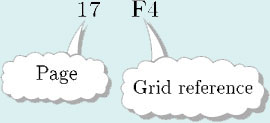To find School Lane on the map, turn to page 17 in the A to Z, move across to column F and then go to row 4:

Similarly, the grid reference for Rickland Place on page 17 in the A to Z is E2.

In mathematics, locating points on a grid is similar to finding a place on a map by means of grid references. However, the grid lines themselves are labelled, rather than the squares. For example, on the grid below, the point A is located by moving across 3 and then up 2.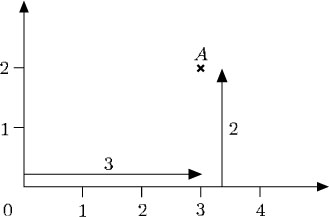In the same way that the grid references on a map are based on a starting point – in Example 8 the starting point was the bottom left corner of the map (column A, row 1) – a starting point is needed to locate points on a mathematical grid. This starting point is called the origin. From the origin you can move horizontally across and vertically up: the line across is called the horizontal axis, and the line going up is called the vertical axis.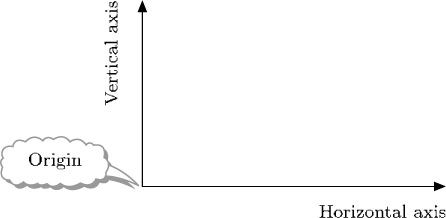On a mathematical grid, the horizontal axis is often labelled the x-axis, and the vertical axis is labelled the y-axis. Scales are indicated on the axes to aid the location of points, and the origin is usually labelled 0.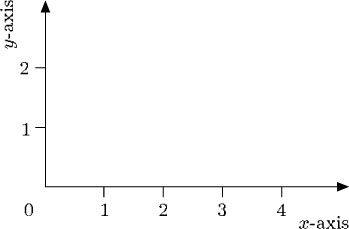The distance across to a point is called the x-coordinate of the point, and the distance up to a point is called the y-coordinate of the point. So, in the example below, A is located at the point with x-coordinate 3 and y-coordinate 2.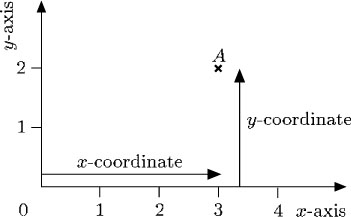The coordinates of a point are written in brackets, with the x-coordinate followed by the y-coordinate, separated by a comma. Thus the coordinates of the point A above are written: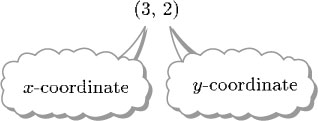### Example 9

Write down the coordinates of the points A and B.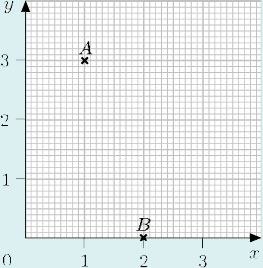To locate A:

• start at the origin;

• move across 1 unit;

• move up 3 units.

The coordinates of A are (1, 3).

To locate B:

• start at the origin;

• move across 2 units;

• move up 0 units.

The coordinates of B are (2, 0).